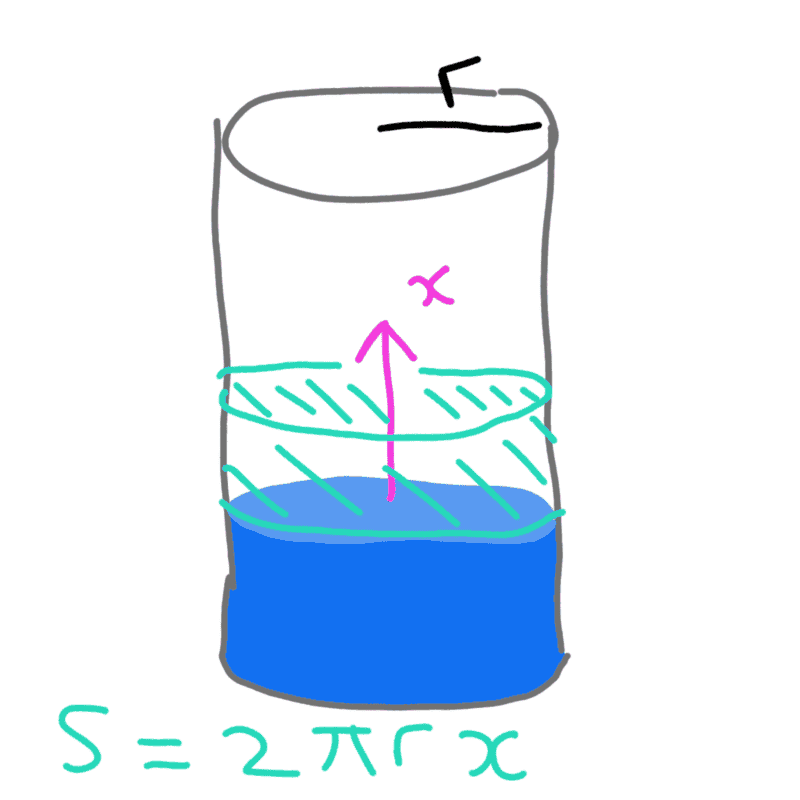# Is this a valid way to calculate capillary force / pressure?

thepopasmurf
When figuring out the capillary pressure on a liquid in a tube of a certain cross-section, the typical approach is to consider the Young-Laplace pressure and the curvature etc.

I was looking through some of my old notes and I had an equation for the capillary force:

fc = γ cosθ dS/dx

where γ is the surface tension,
θ is the contact angle,
S is the surface area to be covered by the liquid (not the cross-sectional surface area, A)
x is the direction of motion of the liquid.

With this equation, I can correctly deduce the capillary pressure in a cylinder (2γ cosθ / r),
and between parallel plates (2γ cosθ / h).

Can this equation be applied to an arbitrary (constant) cross-section?

Usually I see this kind of question tackled with the Young-Laplace equation, but that seems to be complicated for arbitrary cross-sections.

Mentor
Can you please show in a diagram what S and x are?

thepopasmurfDoes this help?

Mentor
thepopasmurf
Yes, that's right

Mentor
These equations are basically the result of a force balance on the meniscus, in the tube case, assuming a spherical segment for the surface, and for the flat channel, assuming a cylindrical segment for the surface (in each case so that the curvature of the surface is constant). The equations do not give the force, but rather the pressure difference across the meniscus surface.

thepopasmurf
Hmm, maybe I should demonstrate what I mean for clarification:

Cylindrical tube: fc = γ cos θ dS/dx,
Surface area in cylinder, S = 2πrx
Cross-sectional area, A = πr2
So Pc = fc/A = 2πrγ cosθ / πr2
Pc = 2γ cosθ / r

Rectangular tube, w*h*x (x is direction of fluid motion):
Surface area in rectangular tube, S = 2wx + 2hx
Cross-sectional area, A = wh
Pc = fc/A = 2*(w + h)γ cosθ / wh = 2γ cosθ (1/h + 1/w)

Which recovers the parallel plate solution when w>>h.

Can this approach be used generally for any constant perimeter / cross-sectional area geometry?

Mentor
Hmm, maybe I should demonstrate what I mean for clarification:

Cylindrical tube: fc = γ cos θ dS/dx,
Surface area in cylinder, S = 2πrx
Cross-sectional area, A = πr2
So Pc = fc/A = 2πrγ cosθ / πr2
Pc = 2γ cosθ / r

Rectangular tube, w*h*x (x is direction of fluid motion):
Surface area in rectangular tube, S = 2wx + 2hx
Cross-sectional area, A = wh
Pc = fc/A = 2*(w + h)γ cosθ / wh = 2γ cosθ (1/h + 1/w)

Which recovers the parallel plate solution when w>>h.

Can this approach be used generally for any constant perimeter / cross-sectional area geometry?
In my judgment, yes, for constant cross section normal to vertical. It is just the integral of the Young-Laplace equation over the surface. However, I do think another constraint has to be that the opening is sufficiently small so that the curvature is approximately constant over the surface. For example, I don't think it is correct for a liquid in a beaker, where the middle area is flat, and the pressure difference across the interface in this area is zero. It would however give the pressure difference averaged over the area.

thepopasmurf
Thank you for your responses and help,